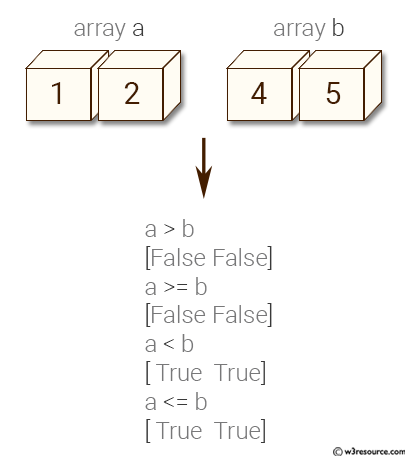﻿ NumPy: Compare two given arrays - w3resource# NumPy: Compare two given arrays

## NumPy: Array Object Exercise-28 with Solution

Write a NumPy program compare two given arrays.

Pictorial Presentation:Sample Solution:

Python Code:

``````import numpy as np
a = np.array([1, 2])
b = np.array([4, 5])
print("Array a: ",a)
print("Array b: ",b)
print("a > b")
print(np.greater(a, b))
print("a >= b")
print(np.greater_equal(a, b))
print("a < b")
print(np.less(a, b))
print("a <= b")
print(np.less_equal(a, b))
```
```

Sample Output:

```Array a:  [1 2]
Array b:  [4 5]
a > b
[False False]
a >= b
[False False]
a < b
[ True  True]
a <= b
[ True  True]
```

Python Code Editor:

Have another way to solve this solution? Contribute your code (and comments) through Disqus.

What is the difficulty level of this exercise?

Test your Python skills with w3resource's quiz

﻿

## Python: Tips of the Day

Generator comprehension:

```# A generator comprehension is the lazy version of a list comprehension.
>>> m = (x ** 2 for x in range(5))
>>> m
<generator object <genexpr> at 0x108efe408>
>>> list(m)
[0, 1, 4, 9, 16]

>>> m = (x ** 2 for x in range(5))
>>> next(m)
0
>>> list(m)
[1, 4, 9, 16]
```